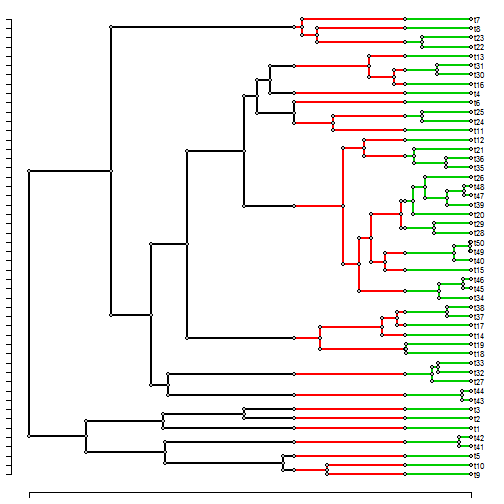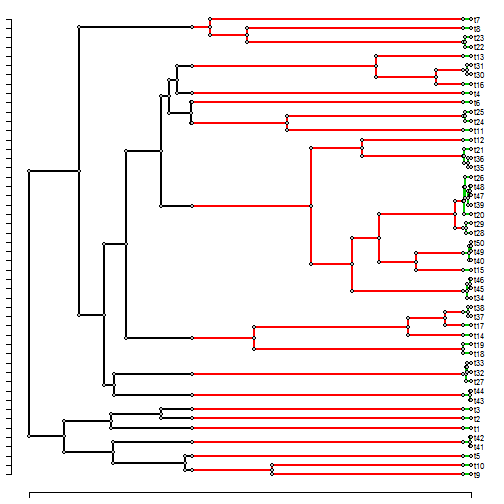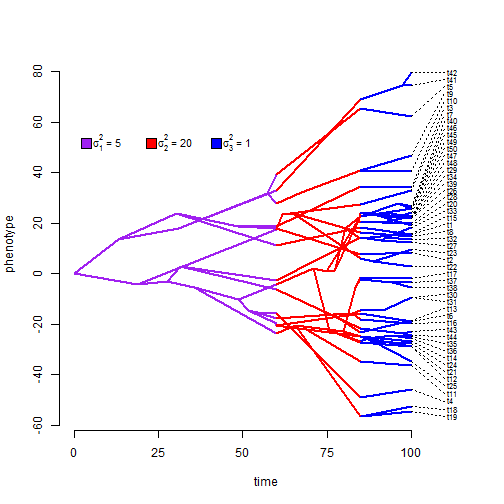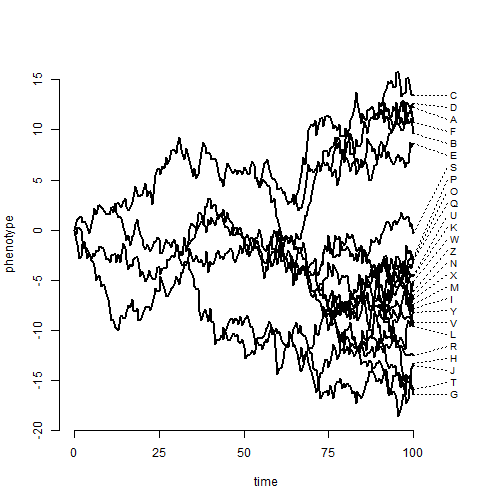## Monday, July 11, 2016

### Using `phenogram` to plot simulated Brownian evolution with multiple rates (and discrete-time BM on trees)

Working on some other stuff, I was wondering how to visualize trait evolution on a tree in which the Brownian rate σ2 is permitted to change along edges of the phylogeny. It is no trouble to simulate tip & node data under this model - because the variance of change is just the sum of each rate multipled by the edge length in the corresponding state. The difficulty in visualizing is that we don't have nodes along those edges at the point where the rate actually changes, so we haven't record the intermediate states. Naturally, then, I thought a sensible framework would be to use trees with singleton nodes. Here's what I mean:

``````library(phytools)
## these are the rates I'm going to simulate:
sig2<-setNames(c(5,20,1),1:3)
## next simulate a tree & data
set.seed(299)
## tree with mapped state corresponding to eras
tree<-make.era.map(pbtree(n=50,scale=100),c(0,60,85))
## convert to tree with singleton nodes:
tmp<-obj<-map.to.singleton(tree)
par(cex=0.7)
plotTree.singletons(obj)
````````````## now simulate by stretching the edges by state
tmp\$edge.length<-tmp\$edge.length*sig2[names(tmp\$edge.length)]
par(cex=0.7)
plotTree.singletons(tmp) ## stretched
````````````x<-fastBM(tmp,internal=TRUE)
``````
``````## now build a maps list for plotting
obj\$maps<-list()
for(i in 1:nrow(obj\$edge)) obj\$maps[[i]]<-setNames(obj\$edge.length[i],
names(obj\$edge.length)[i])
## here is our traitgram
phenogram(obj,x,colors=setNames(c("purple","red","blue"),1:3),
``````
``````## Optimizing the positions of the tip labels...
``````
``````add.simmap.legend(leg=
c(expression(paste(sigma^2," = ",5,sep="")),
expression(paste(sigma^2," = ",20,sep="")),
expression(paste(sigma^2," = ",1,sep=""))),
colors=c("purple","red","blue"),prompt=FALSE,x=5,y=50,
vertical=FALSE,fsize=0.9)
``````Now `fastBM` can simulate up a tree with singleton nodes with no trouble at all, and `phenogram` can obviously plot trees with singleton nodes (as long as it doesn't have to estimate their states); however other function (for instance, `fastAnc`) have a lot more trouble! I'll have to work on that.

It also occurred to me that I could use the same trick to simulate discrete- time Brownian motion on phylogenies as follows:

``````## tree with mapped state corresponding to eras
tree<-make.era.map(pbtree(n=26,tip.label=LETTERS,scale=100),0:200/2)
obj<-map.to.singleton(tree)
x<-fastBM(obj,internal=TRUE)